Next: The spectrum of primordial Up: Describing the inhomogeneities of Previous: The linear approximation

## The Zeldovich approximation

A simple and elegant approximation to describe the non-linear stage of gravitational evolution has been developed by Zeldovich  (see the review by Shandarin & Zeldovich , for an exhaustive description of the Zeldovich approximation). In this approach, the initial matter distribution is considered to be homogeneous and collisionless. If the unperturbed (initial) Lagrangian coordinates of the particles are described by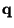, then the Eulerian coordinates of the particles at the time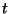are given by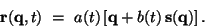(15)

Here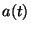is the cosmic expansion factor and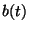the growing rate of linear fluctuations, as provided by eq.(13). Moreover, the velocity term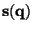, which provides the particle displacement with respect to the initial (Laplacian) position, is related to the potential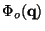originated by the initially linear fluctuations, according to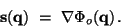(16)

In order to better visualize the meaning of eq.(15), let us consider a pressureless and viscosity-free, homogeneous medium without any gravitational interaction. For this system, the Eulerian positions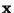of the particles at timeare related to the Lagrangian positionsby the linear relation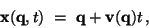(17)

being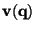the initial velocity. The above expression is essentially analogous to the Zeldovich approximation (15), apart from the presence of theterm, which accounts for the background cosmic expansion, and of theterm, which accounts for the presence of gravity, giving a deceleration of particles along the trajectories (actually,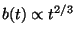in a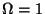matter dominated Universe).

Since at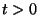density inhomogeneities are created, mass conservation requires that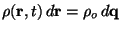, so that the density field as a function of Lagrangian coordinates reads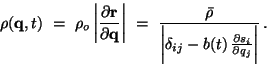(18)

Here the deformation tensor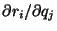accounts for the gravitational evolution of the fluid, while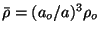is the mean density at time. At the linear stage, when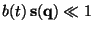, eq.(18) can be approximated by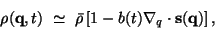(19)

so that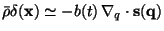and we recover (the growing mode of) the linear solution.

More in general, since the expression (16) formakes the deformation tensor a real symmetric matrix, its eigenvectors define a set of three principal (orthogonal) axes. After diagonalization, eq.(18) can be written in terms of its eigenvalues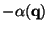,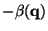and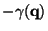, which give the contraction or expansion along the three principal axes:(20)

If the eigenvalues are ordered in such a way that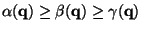, then, asgrows, the first singularity in eq.(20) occurs in correspondence of the Lagrangian coordinate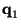, where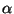attains its maximum positive value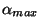, at the time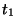such that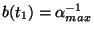. This corresponds to the formation of a pancake (sheet-like structure) by contraction along one of the principal axes. For this reason, Zeldovich  argued that pancakes are the first structures formed by gravitational clustering. Other structures like filaments and knots come from simultaneous contractions along two and three axes, respectively. Doroshkevich  evaluated the probability distribution for the three eigenvalues in the case of a Gaussian random field and concluded that simultaneous vanishing of more than one of them is quite unlikely. Thus, in this scenario, pancakes are the dominant features arising from the first stages of non-linear gravitational clustering.

The Zeldovich approximation predicts the first non-linear structure to arise in correspondence of the high peaks of the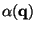field and represents a significant step forward with respect to linear theory and in fact it has been successfully applied to describe the large scale clustering in the distribution of galaxy clusters.

However, within the Zeldovich prescription, after a pancake forms in correspondence of crossing of particle orbits, such particles continue travelling along straight lines, according to eq.(15). Viceversa, in the framework of a realistic description of gravitational dynamics, we expect that the potential wells, that correspond to non-linear structures, should be able to retain particles and to accrete from surrounding regions.Next: The spectrum of primordial Up: Describing the inhomogeneities of Previous: The linear approximation
Waleska Aldana Segura 2001-01-16